2015-10-13 15:51:00 ternenceWei 阅读数 469
• ###### MATLAB图像处理

全面系统的学习MATLAB在图像处理中的应用

19860 人正在学习 去看看 魏伟+=1.首先准备好原图

UIImage* rawImage = [UIImage imageNamed:@"photo.png”];
2.初始化绘图环境
CGSize size = image.size;
UIGraphicsBeginImageContextWithOptions(size, NO, 0);
CGContextRef ctx = UIGraphicsGetCurrentContext();
3.将原图先画入内存中
[image drawInRect:CGRectMake(0,0, size.width, size.height)];
blendMode:kCGBlendModeDestinationIn
alpha:1];
//此处的参数可分为source和destination，分别去共同部分和不同部分。
6.生成想要的结果图
UIImage* retImage = UIGraphicsGetImageFromCurrentImageContext();
UIGraphicsEndImageContext();
return retImage;

ok,会了这招以后，接下来运用到实战当中，比如在QQ的多人聊天组头像（梅花状头像组），就可以通过这种方式来实现。以3个头像为例：
1.先计算出三个头像的分布

if (imageCount == 3) {

}
2.在确定三个头像的位置后，接下来确定各自的角度

for ( int j=0; j<imageCount; ++j ){

UIImage *image_inx = [imageArray objectAtIndex:j];
if (imageCount > 2 || j == 0) {

double angel = startAngel - 2 * j * M_PI / imageCount;

3.然后根据各自度数进行’月牙形’裁剪

}

CGRect rcImage = [[rectArray objectAtIndex:j] CGRectValue];
CGContextDrawImage(context, rcImage, image_inx.CGImage);
}
4.最后将三幅图都画在一起便得到了一个梅花状的头像组。

2019-05-15 23:00:39 qq_38024084 阅读数 480
• ###### MATLAB图像处理

全面系统的学习MATLAB在图像处理中的应用

19860 人正在学习 去看看 魏伟

# 灰度变换

## 灰度变换  空间滤波

### 图像反转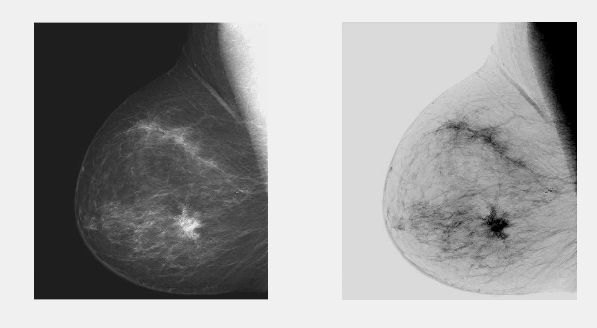imadjust（f,[low_in, high_in ],[low_out, high_out]）将图片的[low_in, high_in]范围映射到[low_out, high_out]，我们需要进行反转，就将low_out > low_in

f=imread('C:\Matlab Project\image\Fig0303(a)(breast).tif');
figure(1)
title('图像反转');
subplot(1,2,1);imshow(f)
subplot(1,2,2);imshow(g)


### 对数变换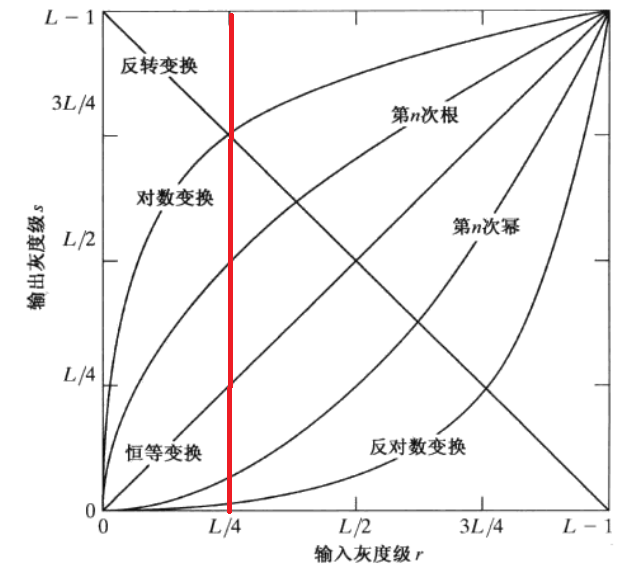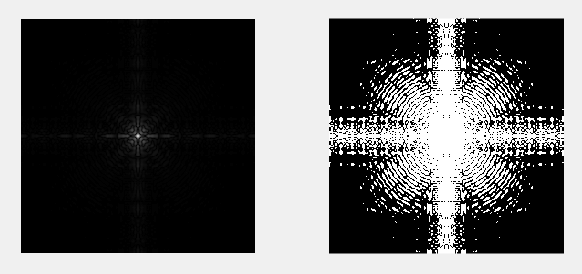f=imread('C:\Matlab Project\image\Fig0305(a)(spectrum).tif');
g=0.8*log(1+double(f));
figure()
subplot(1,2,1);imshow(f)
subplot(1,2,2);imshow(g)

### 幂次变换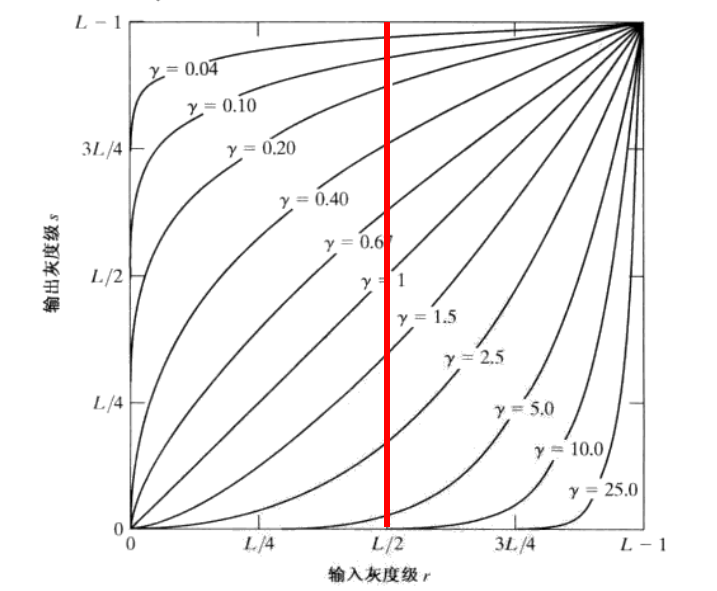<1时，效果和对数函数相似，放大暗处细节，压缩亮处细节，随着数值减少，效果越强

>1时，放大亮处细节，压缩暗处细节，随着数值增大，效果越强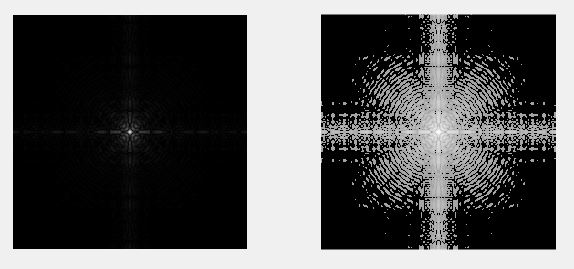f=imread('C:\Matlab Project\image\Fig0305(a)(spectrum).tif');
g=double(f).^0.1;
figure()
subplot(1,2,1);imshow(f,[])
subplot(1,2,2);imshow(g,[])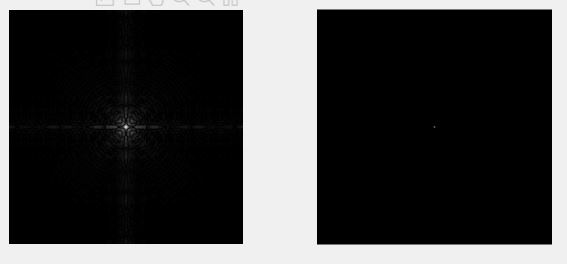f=imread('C:\Matlab Project\image\Fig0305(a)(spectrum).tif');
g=double(f).^10;
figure()
subplot(1,2,1);imshow(f,[])
subplot(1,2,2);imshow(g,[])

### 位图切割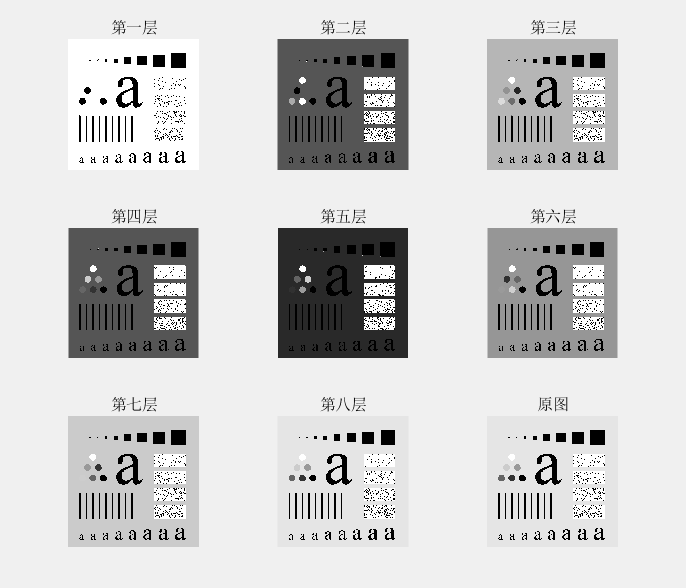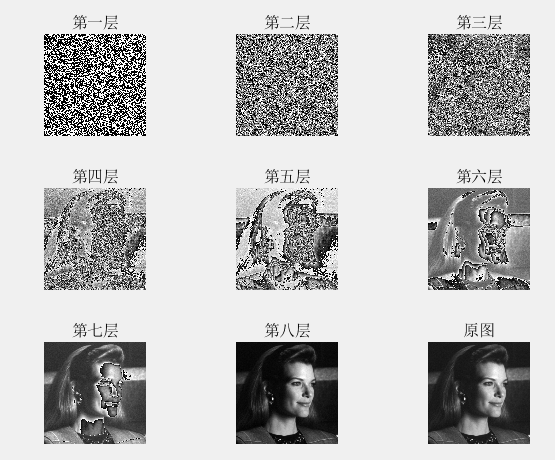f=imread('C:\Matlab Project\image\original_test_pattern.tif');
g=double(f);
L1=mod(g,2);
L2=mat2gray(mod(g./2,2));
L3=mat2gray(mod(g./(2.^2),2));
L4=mat2gray(mod(g./(2.^3),2));
L5=mat2gray(mod(g./(2.^4),2));
L6=mat2gray(mod(g./(2.^5),2));
L7=mat2gray(mod(g./(2.^6),2));
L8=mat2gray(mod(g./(2.^7),2));
figure()
subplot(3,3,1);imshow(L1),title('第一层');
subplot(3,3,2);imshow(L2),title('第二层');
subplot(3,3,3);imshow(L3),title('第三层');
subplot(3,3,4);imshow(L4),title('第四层');
subplot(3,3,5);imshow(L5),title('第五层');
subplot(3,3,6);imshow(L6),title('第六层');
subplot(3,3,7);imshow(L7),title('第七层');
subplot(3,3,8);imshow(L8),title('第八层');
subplot(3,3,9);imshow(f),title('原图');

### 直方图：

表示像素值在图像中出现的次数，下图显示的是图片对应的直方图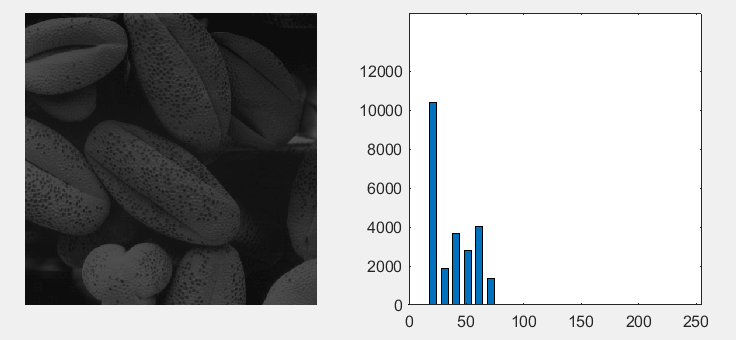f=imread('C:\Matlab Project\image\Fig0308(a)(pollen).tif');
h1=imhist(f,256); %函数imhist 得到一维数组频数 不直接输出
h2=h1(1:10:256); %转化为条形图
horz=1:10:256;
figure()
subplot(1,2,1);imshow(f);
subplot(1,2,2); h2=bar(horz,h2,0.6);
axis([0 255 0 15000]) %直方图输出范围（横0-256 纵 0-15000 ）
set(gca,'xtick',0:50:255)
set(gca,'ytick',0:2000:12000)

### 直方图均衡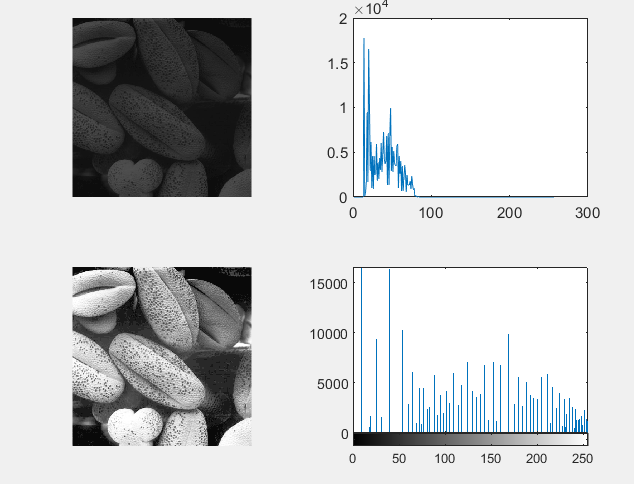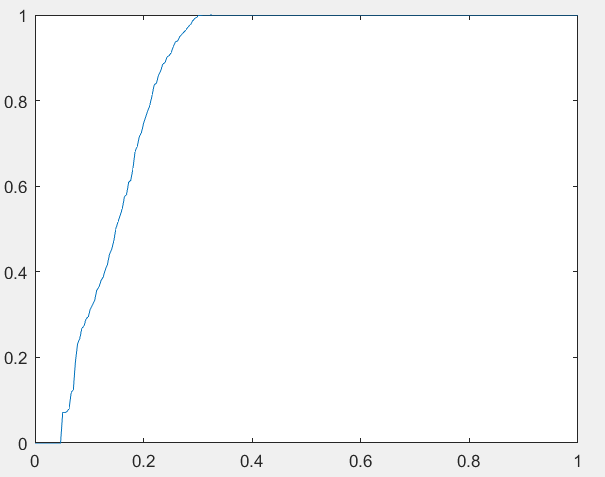matlab 中使用histeq(f,256)直接获得均衡后的图像

f=imread('C:\Matlab Project\image\Fig0308(a)(pollen).tif');
h1=imhist(f,256);
h2=histeq(f,256);  %histeq均衡后得到为图像
figure(1)
subplot(2,2,1);imshow(f);
subplot(2,2,2);plot(h1);
subplot(2,2,3);imshow(h2);
subplot(2,2,4);imhist(h2);

hnorm=imhist(f)./numel(f);  %注意为原图像的概率，不是均衡后的
cdf=cumsum(hnorm);  % 变换函数S=T（r）
x=linspace(0,1,256);
figure(2)
plot(x,cdf);
axis([0 1 0 1])
set(gca,'xtick',0:0.2:1)
set(gca,'ytick',0:0.2:1)

### 直方图匹配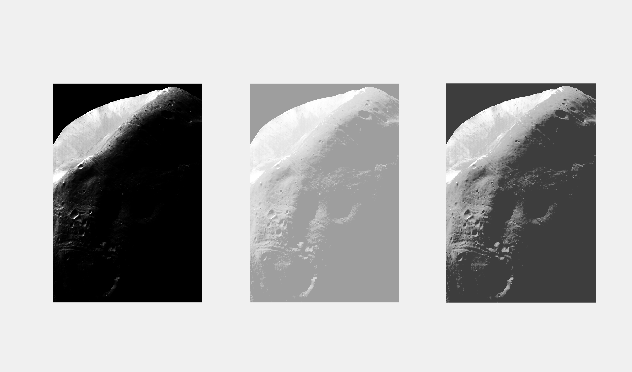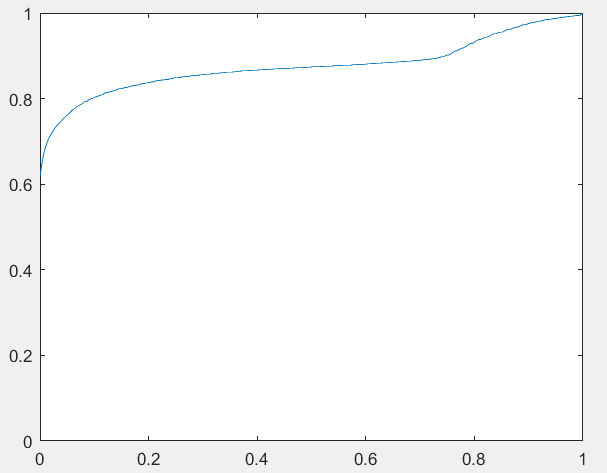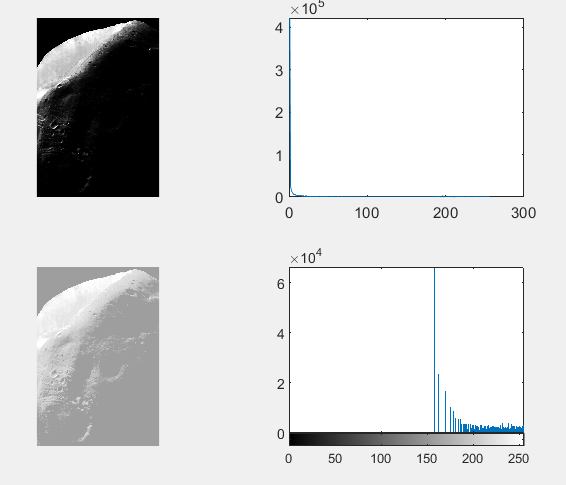matlab 通过  histeq(f,hspec) 函数来实现，hspec为最后希望的直方图

twomodegauss

function p = twomodegauss(m1,sig1,m2,sig2,A1,A2,k)
%TWOMODEGAUSS 返回双峰高斯函数
%   p = twomodegauss(m1,sig1,m2,sig2,A1,A2,k)
%   ｐ为标准化２５６个元素，ｓｕｍ（ｐ）＝１，
% 　（ｍ１，ｓｉｇ１），（ｍ２，ｓｉｇ２）分别为双峰的均值和标准差，
% 　Ａ１，Ａ２分别为峰值，ｋ为基数

c1 = A1 * (1 / ((2 * pi) ^ 0.5) * sig1);
k1 = 2 * (sig1 ^ 2);
c2 = A2 * (1 / ((2 * pi) ^ 0.5) * sig2);
k2 = 2 * (sig2 ^ 2);
z = linspace(0, 1, 256);

p = k+c1*exp(-((z-m1).^2)./k1)+c2*exp(-((z-m2).^2)./k2);
p = p./sum(p(:));


f=imread('C:\Matlab Project\image\Fig0310(a)(Moon Phobos).tif');
p = twomodegauss(0.15,0.05, 0.75, 0.05,1, 0.07, 0.002);
g=histeq(f,p);
figure(1)
subplot(1,3,1);imshow(f);
subplot(1,3,2);imshow(histeq(f,256));
subplot(1,3,3);imshow(g);


2018-03-28 16:28:10 qq_38784454 阅读数 1718
• ###### MATLAB图像处理

全面系统的学习MATLAB在图像处理中的应用

19860 人正在学习 去看看 魏伟
clc;
clear all;
[pathname,filename] = uigetfile('*.*','选择图片','E:\裂纹\NG2裂纹\');
src_path =strcat(filename,pathname) ;
dst_path = 'E:\学习资料\MATLAB程序\裂纹分割\';
if ~exist('E:\学习资料\MATLAB程序\裂纹分割\')
mkdir('E:\学习资料\MATLAB程序\裂纹分割\');
end

figure;
imshow(A);
[m,n,l] = size(A);
a = 8;
figure;
for i = 1:a
for j = 1:a
m_start = 1+ (i-1)*fix(m/a);
m_end = i*fix(m/a);
n_start = 1+ (j-1)*fix(m/a) ;
n_end = j*fix(n/a);
AA = A(m_start:m_end,n_start:n_end,:);
imwrite(AA,[dst_path num2str(i) '_' num2str(j) '.jpg'],'jpg');
subplot(8,8,(i-1)*8+j);
imshow(AA);
hold on;
end
end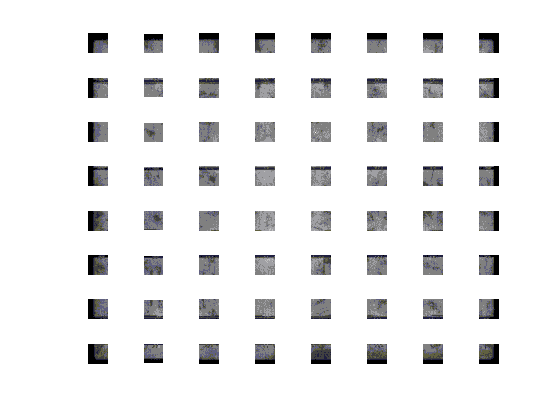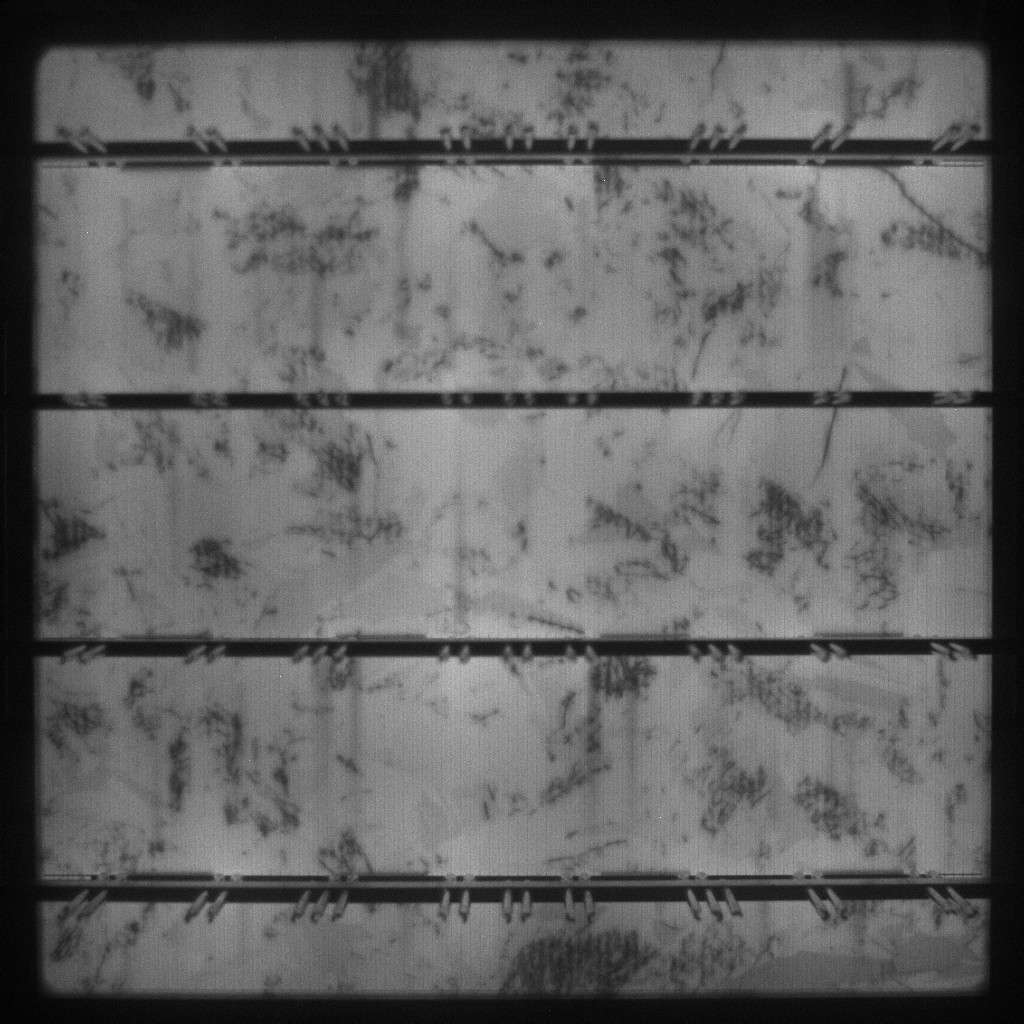2014-10-12 13:25:40 wozhendebuhaoma 阅读数 2759
• ###### MATLAB图像处理

全面系统的学习MATLAB在图像处理中的应用

19860 人正在学习 去看看 魏伟

http://zhidao.baidu.com/question/519528446090102645.html

http://zhidao.baidu.com/question/391593318895377005.html

http://zhidao.baidu.com/question/617082845639831412.html

http://zhidao.baidu.com/question/1946820750805557828.html

http://zhidao.baidu.com/question/1702286530212076180.html

http://zhidao.baidu.com/question/1238528588771891699.html

http://zhidao.baidu.com/question/1238528652585891459.html

http://zhidao.baidu.com/question/2010245200610808468.html

http://zhidao.baidu.com/question/2010308752180544028.html

http://zhidao.baidu.com/question/1238592204736973419.html

http://zhidao.baidu.com/question/2010309201259490868.html

http://zhidao.baidu.com/question/680698400065462692.html

http://zhidao.baidu.com/question/455208937458346285.html

http://zhidao.baidu.com/question/1860437354781339187.html

http://zhidao.baidu.com/question/1238656589677680619.html

http://zhidao.baidu.com/question/1238656653749463099.html

http://zhidao.baidu.com/question/680698721128169452.html

http://zhidao.baidu.com/question/1860437611195301507.html

http://zhidao.baidu.com/question/1860437674878353067.html

2019-12-20 10:36:34 rong11417 阅读数 141
• ###### MATLAB图像处理

全面系统的学习MATLAB在图像处理中的应用

19860 人正在学习 去看看 魏伟

1.介绍

2.模拟图像处理

3.数字图像处理

4.什么是图像

5.数字图像和信号之间的关系

6.如何形成数字图像

7.应用

## 4.什么是图像128 30 123 232 123 321 123 77 89 80 255 255

## 7.应用

### 机器/计算机视觉### 计算机图形学